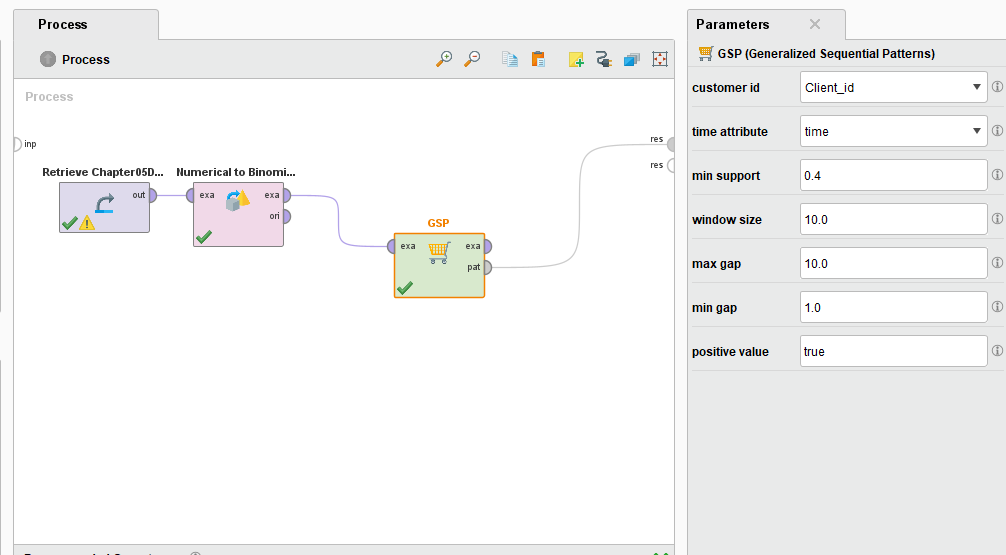# Generalized Sequential Pattern (GSP)

Member Posts: 1Contributor I
edited November 2018 in Help

My name is Tasos Ioannou and I am a Phd student from TU Delft, the Netherlands.

I am new to rapid miner and I am trying to play with GSP in order to find patterns of occupancy (daily presence or not) in residential houses.

My data are like this:

Timestamp      Type of Room--    House 1 --  House 2 -- House 3 -- etc.
3/6/2015 00:00      Kitchen                 0                   1                1
3/6/2015 00:05      Kitchen                 1                   0                1
3/6/2015 00:10      Kitchen                 0                   1                1
3/6/2015 00:20      Kitchen                 0                   0                0

So first column is the time stamp (every five minutes for a period of several months), second column is the type of room and the rest of the columns are the readings of the presence sensors in 0,1 format (1 when a person's presence was detected within the five minutes interval and 0 when no presence was detected).

I am trying to use the GSP to find patterns of occupancy for a whole day between all the houses (32 dwellings in total). Following the description of the process operator and looking at the tutorial example I have made a file but seems that  I am missing something since instead of results I am getting a view of the example set (!) which I have already seen before using the ''break point after'' option.

The customer id is the type of rooms (Kitchen, Living Room etc), the houses (House 1, House 2 etc) are the attributes.

My questions are as follows:

1) For the time attribute I am transforming the date to numerical as necessary, that would result in a time column from 1-288. Does that make sense? In the tutorial example the time is a column with only one value (1).

2) Do you think there is maybe another problem? Maybe the GSP is not the correct tool for what I am trying to achieve? I would really use some suggestions on this, on how to improve my set up, or use another process operator?

Note that I have made all the necessary transformations to the data (the 0,1 have been transformed into true or false)

The results I was hoping could be described like this: in specific 5 minute intervals of the day, lets say 6/3/2015 15:55 there is presence detected in (House1,House 2,House3, House4 etc). Like that I was hoping to identify the times of the day where most of the houses have occupancy detected or not.

The code for the  whole process:

<?xml version="1.0" encoding="UTF-8" standalone="no"?>
<process version="6.5.002">
<context>
<input/>
<output/>
<macros/>
</context>
<operator activated="true" class="process" compatibility="6.5.002" expanded="true" name="Process">
<process expanded="true">
<parameter key="excel_file" value="D:\Ecommon Data\Data Analysis\Houses without Balanced Ventilation\Yes-No\Presence.xlsx"/>
<parameter key="sheet_number" value="5"/>
<parameter key="imported_cell_range" value="A1:L289"/>
<parameter key="first_row_as_names" value="false"/>
<list key="annotations">
<parameter key="0" value="Name"/>
</list>
<list key="data_set_meta_data_information">
<parameter key="0" value="Customer id.true.polynominal.attribute"/>
<parameter key="1" value="W001.true.integer.attribute"/>
<parameter key="2" value="W002.true.integer.attribute"/>
<parameter key="3" value="W010.true.integer.attribute"/>
<parameter key="4" value="W011.true.integer.attribute"/>
<parameter key="5" value="W021.true.integer.attribute"/>
<parameter key="6" value="W022.true.integer.attribute"/>
<parameter key="7" value="W024.true.integer.attribute"/>
<parameter key="8" value="W028.true.integer.attribute"/>
<parameter key="9" value="W032.true.integer.attribute"/>
<parameter key="10" value="Time.true.date_time.attribute"/>
<parameter key="11" value="L.false.attribute_value.attribute"/>
</list>
</operator>
<operator activated="true" breakpoints="after" class="date_to_numerical" compatibility="6.5.002" expanded="true" height="76" name="Date to Numerical" width="90" x="246" y="75">
<parameter key="attribute_name" value="Time"/>
<parameter key="time_unit" value="minute"/>
<parameter key="minute_relative_to" value="day"/>
</operator>
<operator activated="true" breakpoints="after" class="numerical_to_binominal" compatibility="6.5.002" expanded="true" height="76" name="Numerical to Binominal" width="90" x="380" y="75">
<parameter key="attribute_filter_type" value="subset"/>
<parameter key="attributes" value="W001|W002|W010|W011|W021|W022|W024|W028|W032"/>
</operator>
<operator activated="true" class="generalized_sequential_patterns" compatibility="6.5.002" expanded="true" height="76" name="GSP" width="90" x="581" y="75">
<parameter key="customer_id" value="Customer id"/>
<parameter key="time_attribute" value="Time"/>
<parameter key="window_size" value="1.0"/>
<parameter key="max_gap" value="1.0"/>
<parameter key="min_gap" value="1.0"/>
<parameter key="positive_value" value="true"/>
</operator>
<connect from_op="Read Excel" from_port="output" to_op="Date to Numerical" to_port="example set input"/>
<connect from_op="Date to Numerical" from_port="example set output" to_op="Numerical to Binominal" to_port="example set input"/>
<connect from_op="Numerical to Binominal" from_port="example set output" to_op="GSP" to_port="example set"/>
<connect from_op="GSP" from_port="example set" to_port="result 1"/>
<portSpacing port="source_input 1" spacing="0"/>
<portSpacing port="sink_result 1" spacing="0"/>
<portSpacing port="sink_result 2" spacing="0"/>
</process>
</operator>
</process>

I am looking forward to hearing from you, thank you in advance for your time and effort on this.

Kind Regards
Tasos Ioannou

• Member Posts: 1Newbie
Hi,
DataSet for GSP
Client_id, time , feature 1, feature 2, feature 3
1,1,0,1,0
1,2,1,1,1
1,3,0,1,0
1,4,1,1,1
2,1,0,1,0
2,2,1,1,1
2,3,0,1,0
2,4,1,1,1
3,1,0,1,0
3,2,1,1,1
3,3,0,1,0
3,4,1,1,1
4,1,0,1,0
4,2,1,1,1
4,3,0,1,0
4,4,1,1,1